Next: K-L algorithm Up: Graph Theory Based Algorithms Previous: Greedy algorithm

### Spectral bisection

The recursive spectral bisection (RSB) algorithm [68,75,87] is based on the following consideration. Let each vertex of the graph be assigned a value of either 1 or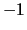, and let the value assigned to vertex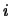be denoted by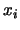. This creates a bisection of the graph in which the vertices having value 1 form one subdomain and those with valueform the other. The edge-cut for the bisection can be expressed as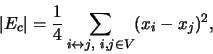(2)

where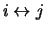means that there is an edge connecting the verticesand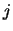. In order to keep the load balanced, each of the two subdomains is required to have the same number of vertices. Thus the sum of all the values associated with the vertices is zero, that is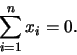(3)

The graph bisection problem is equivalent to minimizing (2) subject to (3) withtaking the value of either 1 or. This is an integer programming problem which is difficult to solve when the number of vertices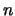is large. Ignoring the integer constraints, the quadratic (2) can be minimized subject to the linear constraint (3) only. This gives a continuous minimization problem. But without an extra constraint the solution is simply the vector of zeros. Remembering that when all the variables take the value 1 or, the sum of the squares should be, the number of vertices. This gives the extra constraint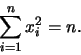(4)

The quadratic (2) can now be minimized subject to (3) and (4).

The quadratic (2) can be written as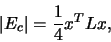(5)

where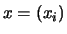is the vector composed of all the values to be assigned to vertices and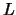is an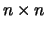matrix known as the Laplacian matrix of the graph. It has the simple form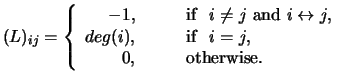(6)

Here deg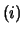is the degree of the vertex, defined in Section 2 as the number of edges connected with the vertex. The matrixsatisfies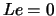with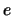the vector of all ones. Applying the necessary condition for the constrained minimization problem gives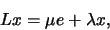(7)

with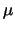and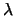two Lagrange multipliers to be decided. Multiplying both sides of the equation by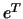gives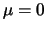. Thus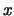has to be an eigenvector ofand the edge-cut is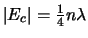. In order to minimize the edge-cut,needs to be the eigenvector of the smallest possible eigenvalue ofthat satisfies the two constraints (3) and (4).

The matrixis positive semi-definite with smallest eigenvalue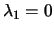and corresponding eigenvector. Clearlyis not a solution to the problem since it does not satisfy the load balancing constraint (3). If the graph is connected, then it can be shown that the next smallest eigenvalue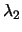is positive . The eigenvector associated with this eigenvalue satisfies (3) because it is orthogonal to the first eigenvector. It also satisfies (4) by proper scaling, and thus is the solution of the constrained minimization problem. This eigenvector (also called the Fiedler vector) gives a value to each vertex of the graph, and the graph can be bisected by separating those with smaller values from those with larger values. The procedure can then be repeated on each of the subdomains. This gives the RSB algorithm. In practice the RSB algorithm almost always gives connected subdomains if the original graph is connected, however there can be exceptions, as Figure 5 shows.

Figure 6 gives a simple graph and the eigenvector associated with the second smallest eigenvalue of the Laplacian. Here the values of the eigenvector are marked beside each vertex in brackets. Sorting the vertices based on the values of the eigenvector bisects the graph into two triangles, with the edge-cut of one.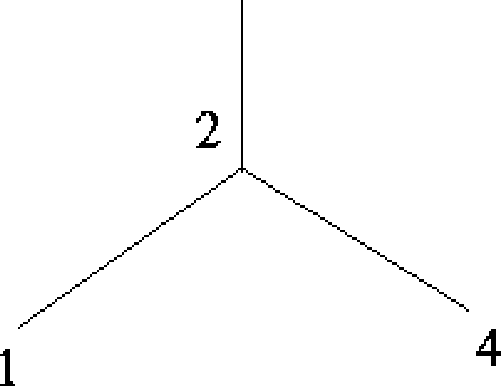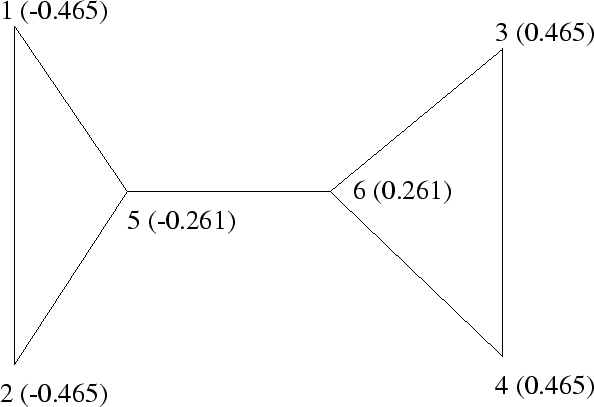To find the eigenvector corresponding to the second smallest eigenvalue, the Lanczos algorithm can be employed. This is an iterative algorithm which requires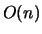operations per iteration. The algorithm normally converges in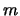iterations, withtypically less than a few hundred. Thus the algorithm has a complexity of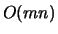. However the Lanczos algorithm requires the storage ofLanczos vectors of length, unless the entire Lanczos recurrence is run through a second time in order to accumulate the eigenvector. An alternative algorithm  for finding the eigenvector is to solve the minimization problem (2) using the conjugate gradient algorithm, which requires the storage of only four vectors. This algorithm was only tested on regular grids.

Figure 7 shows the result of applying the RSB algorithm to the dual graph of the mesh with 788 elements, the partition has 108 shared edges, significantly fewer than the edge-cut of 142 generated by the RCB and RGB algorithms, although the RSB algorithm is far more expensive. In this case all of the subdomains are connected.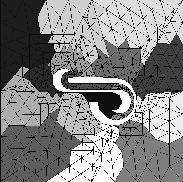Two recursive spectral bisections do not necessarily generate an optimal quadri-section . Direct quadri-section, even octa-section, using two or three eigenvectors of the Laplacian matrix has been explored . Another interesting way of utilizing multiple eigenvectors is to treat them as spectral coordinates of the vertices. Using these coordinates in the context of inertia bisection can generate partitions of better quality than RSB .Next: K-L algorithm Up: Graph Theory Based Algorithms Previous: Greedy algorithm

2000-03-21